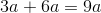# ISEE Middle Level Math : How to add variables

## Example Questions

### Example Question #1 : Expressions & Equations

Simplify the following expression: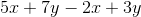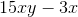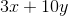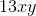Cannot be computedExplanation:

When adding and subtracting variable, you can only combine like variables.

That means all of thevariables are solved separately from thevariables.

Then you just add and subtract the constants normally so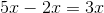and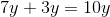.

So the final answer is.

### Example Question #58 : Operations

Simplify: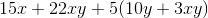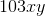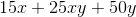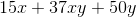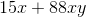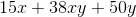Explanation:

Begin by distributing theto its entire group: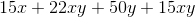Next, group the like terms: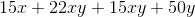Finally, combine the like terms:### Example Question #171 : Algebraic Concepts

When adding variables, we must add all of the like variables together but then combine them into one singular value at the end. True or False

False

True

False

Explanation:

When adding variables, it is true that you must first add all of the like variables.  But then they are left separate to have an expression with the different variables differentiated.

### Example Question #3 : Expressions & Equations

Simplify the followng: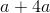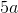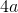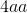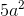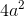Explanation:

When adding variables together, you must first make sure you are combining the same variable.  So, in this casewe can see that both terms contain the variable a.  Therefore, we can combine them.

Now, when we combine them, we can think of the variables as objects.  So, we can say were are combining an apple and 4 apples together.  So,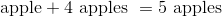We can simplify our problem the same way.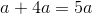### Example Question #31 : How To Add Variables

Combine the following variables: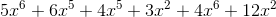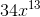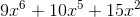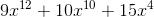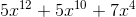Explanation:

Combine the following variables:First, group the like terms. This means we will put terms with the same exponent next to each other. This will make it easier to be sure we are adding correctly.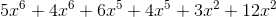Next, add the coefficients, but keep the exponents the same. Only combine terms with the same exponents. Notice that we have three different exponents (6,5 and 2) This means we will have three terms in our final answer.

So we get the following:### Example Question #32 : How To Add Variables

Simplify the following: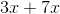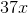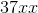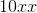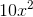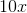Explanation:

To add variables, we will think of the variables as objects.  So, in the problemlet's think of the variable x as books.  So, we can look at the problem as we currently have 3 books.  We go to the library and borrow 7 more books.  How many books do we have now?  The answer is 10.  We now have 10 books.

We can add the variables in the same way.  So,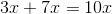### Example Question #33 : How To Add Variables

Simplify the following: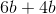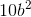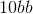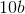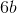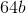Explanation:

To add variables, we will look at the variables as objects.  So,we can look at it as the following.  We have 6 dollars.  We do all of our chores, and we get 4 more dollars.  How many dollars do we have now?  We now have 10 dollars.  We add variables in the same way. So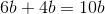### Example Question #34 : How To Add Variables

Simplify the following: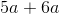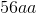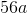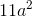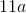Explanation:

When we add variables, we can think of the variables as objects.  So in the problem,we can think of the variable a as apples.  We can say that we currently have 5 apples.  We go to the store and buy 6 more apples.  How many apples do we have now?  We have 11 apples.

We can add variables the same way.  So,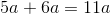### Example Question #35 : How To Add Variables

Combine the following terms.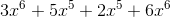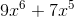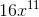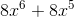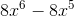Explanation:

Combine the following terms.We need to realize that we can only add variables together if they have the same exponent. Then, all we need to do is add the coefficients (numbers in front)

So, let's reorganize our statement to have variables of the same power together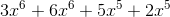Now, we can add the first two and last two to get our answer### Example Question #36 : How To Add Variables

Combine the following: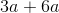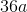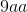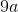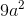Explanation:

To add variables, we will look at the variables like objects.  So in the problemwe will think of a as being apples.  So we can read this problem as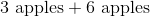So, if we have 3 apples, and we go to the market and we buy 6 more apples, how many apples do we now have?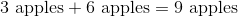We add variables the same way.  So,We can look at this as we have 3 of something, we want to add 6 more somethings, so how many do we have now?